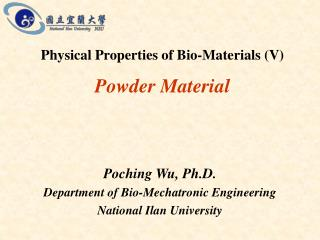DownloadDownload PresentationPowder Material

# Powder Material

Télécharger la présentation## Powder Material

- - - - - - - - - - - - - - - - - - - - - - - - - - - E N D - - - - - - - - - - - - - - - - - - - - - - - - - - -
##### Presentation Transcript

1. Powder Material Physical Properties of Bio-Materials (V) Poching Wu, Ph.D. Department of Bio-Mechatronic Engineering National Ilan University

2. Particles Characteristics • Shape • Mean Particle Size • Density • Surface Activity • Mechanical Strength • Dust Formation

3. Fineness Modulus & Average Diameter

4. Geometric Mean Diameter • dgw = geometric mean diameter, m • di = diameter of sieve opening of the i’th sieve, m • di+1= diameter of opening in next larger than i’th sieve (just above in a set), m • di= geometric mean diameter of particles on I’th sieve, m • Wi = weight fraction on i’th sieve

5. Geometric Standard deviation • Sgw = geometric standard deviation, m

6. Equivalent Spherical DiameterStokes’ Diameter, de where  = viscosity, poise p = sample density, g/cm3 l = fluid density, g/cm3 g = gravity, 980 cm/sec2 h = Height, cm t = time, sec